# Functions

Functions are statements that are defined to perform a particular task. The user can pass arguments into the function and these tasks will be performed on your input. You can define a function and call it multiple times for different inputs. This greatly reduces redundancy and increases the readability of your code.

## Defining and Using a Function

To understand the syntax of defining a function, let’s try out a simple example.

### Example:

This function converts centimeters to inches:

```cm_to_in <- function(cm) {
inch <- (cm/2.54)
return(inch)
}
cm_to_in(5)

```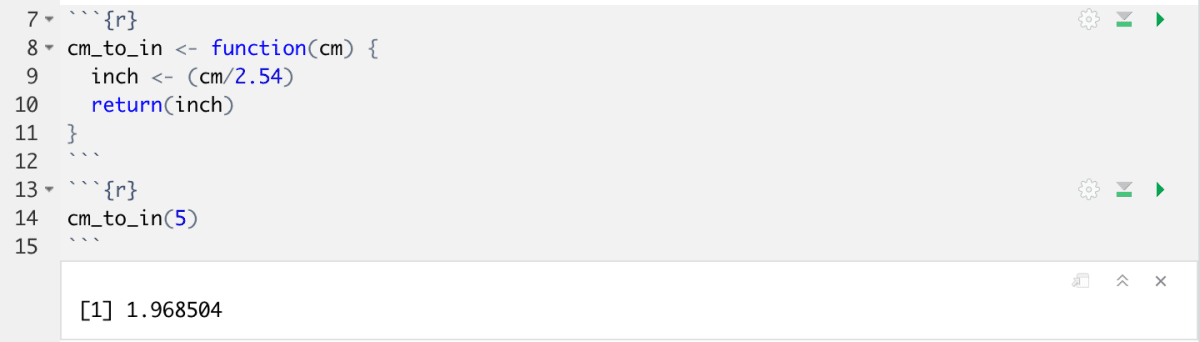### Function Definition

• To define a function the command `function()` is used. The argument the function takes is specified within the brackets, in this case `cm`
• The name of the function is found on the right side of the assignment operation: `cm_to_in`
• The operations of the function are found within the pair of curly brackets`{}`
• The operations can be performed on the arguments that are taken in to the function. This function takes a single argument, `cm` and divides it by 2.54. This is assigned to a new variable, `inch`, and the value of inch is returned as the output.

### Function Call

• To use the function you must call its name `cm_to_in` and give it the required argument or arguments. In this case the function take a single argument, `5`. Any input that we feed the function will be interpreted to be `cm` . The input given should reflect the nature of the function defined. For example, in this case a single integer or floating point number is suitable.
• The output is printed as a result of the function being carried out.

## Built-in Functions:

R and the various packages available for R have a large collection of built-in functions as well.

### Example:

In this example, let us try to use an existing function: seq().

The seq function makes regular sequences of numbers. The syntax is as follows: `seq(from, to)`

Let’s give the arguments `1, 10`. We’ll assign this value to a variable and call the variable to print the output.

```a <- seq(1,10)
a``````b <- seq(1,10, length.out =5)
b```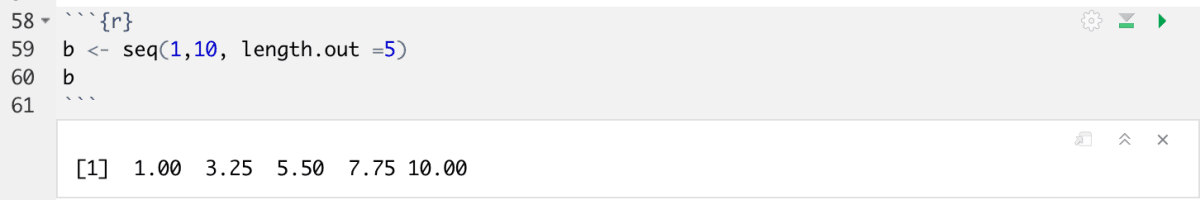The length.out argument modifies the seq() function in the following way: The values from the starting point until the ending point are divided into equal sections based on the number specified (i.e. 1-10 is divided into 5 sections) and the sequence is printed.

In the second example, I used the same function, but I added an extra argument. Functions will require some arguments as mandatory arguments, and some that are optional. The seq() function requires the starting and ending point of the sequence, mandatorily. The length.out argument is optional. Leaving out a required argument would result in an error.

Now look at your environment in the upper right pane: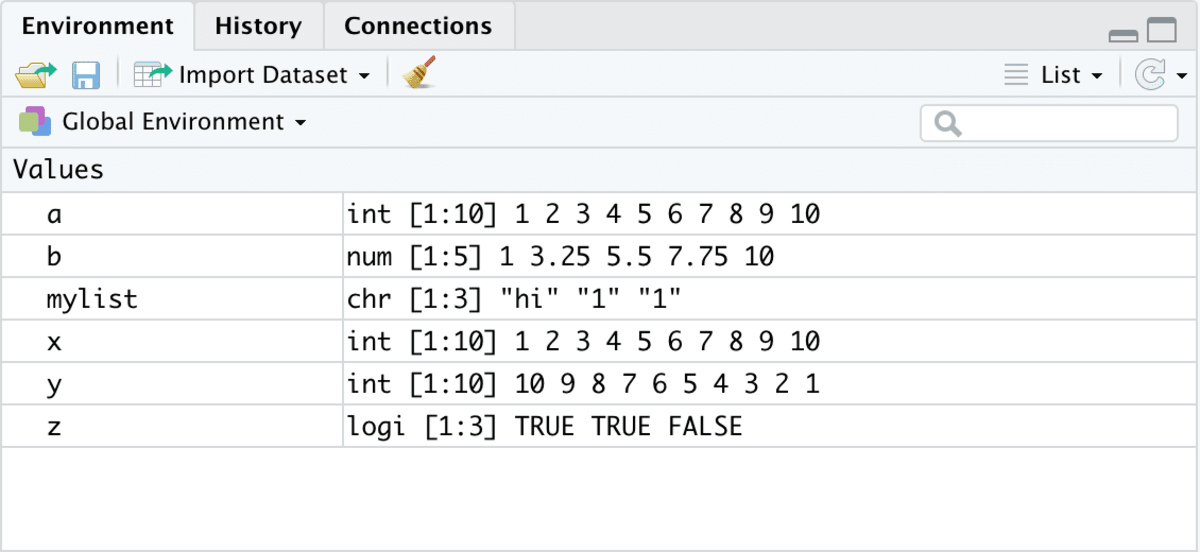Here you can see all of the objects that you’ve created.

## Learning Through Example: Commonly Used Functions

Let’s apply some commonly used functions to a dataset and understand their applications. In this example we are going to be looking at `birthwt` data from the `MASS` package.

Loading in the required libraries and taking a look at the data:

```library(MASS)
library(dplyr)
birthwt```

The data contains information about the birthweight of 189 children, the age of their mothers, and smoking status of the mothers, among other information.

### Filter()

The filter function allows you to select relevant data from a large dataset. In this example, I am subsetting the dataset based on the age of the mother and storing them in separate locations.

```younger <- filter(birthwt, age <= 25)
older <- filter(birthwt, age > 25)

```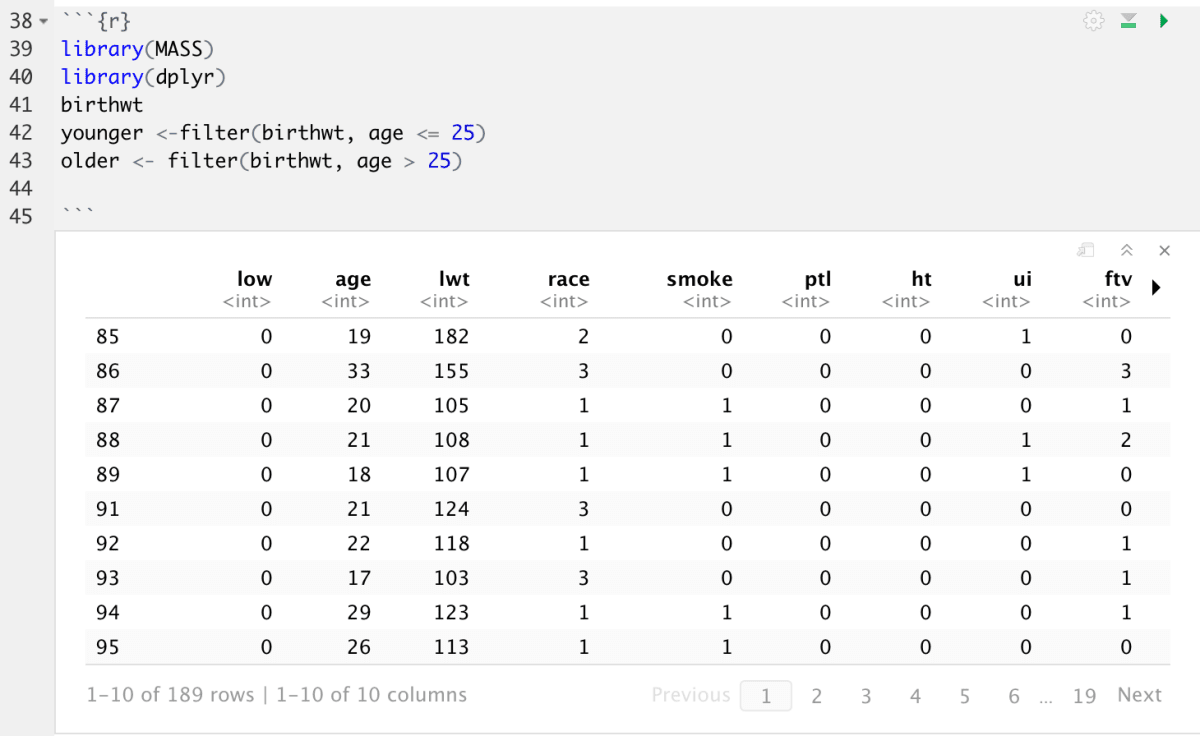The filter function can also be used with multiple conditions. I’ve done a similar operation, using multiple conditions, separated by an or operator.

```younger2 <- filter(birthwt, age > 25 | age == 25)
younger2
```Whenever you start using complicated, multipart expressions in filter(), consider making them explicit variables instead. That makes it much easier to check your work. You’ll learn how to create new variables shortly.

### Grouped summaries with `summarise()`

The next key verb is `summarise()`. It collapses a data frame to a single row. The output is reflective of the entire data it is give. Cool! But I don’t know how helpful a single mean is to me. Pairing this function with the `group_by()` function, which splits the data into groups, drastically improves the scope of the summarise function. Looking at our birthwt data:

`summarise(birthwt, birthweight = mean(bwt, na.rm = TRUE))`We are returned a single summary value telling us the mean is 2944.587 mg. This doesn’t really tell us much. Let’s try the group_by() function now. We begin by grouping the data by a couple of parameters (smoking status and race). Applying the summarize function to the groups returns the summary of the birthweight for each of these groups.

```grouping <- group_by(birthwt, smoke, race)
summarise(grouping, birthweight = mean(bwt, na.rm = TRUE))
bw_sum <- summarise(grouping, birthweight = mean(bwt, na.rm = TRUE))

```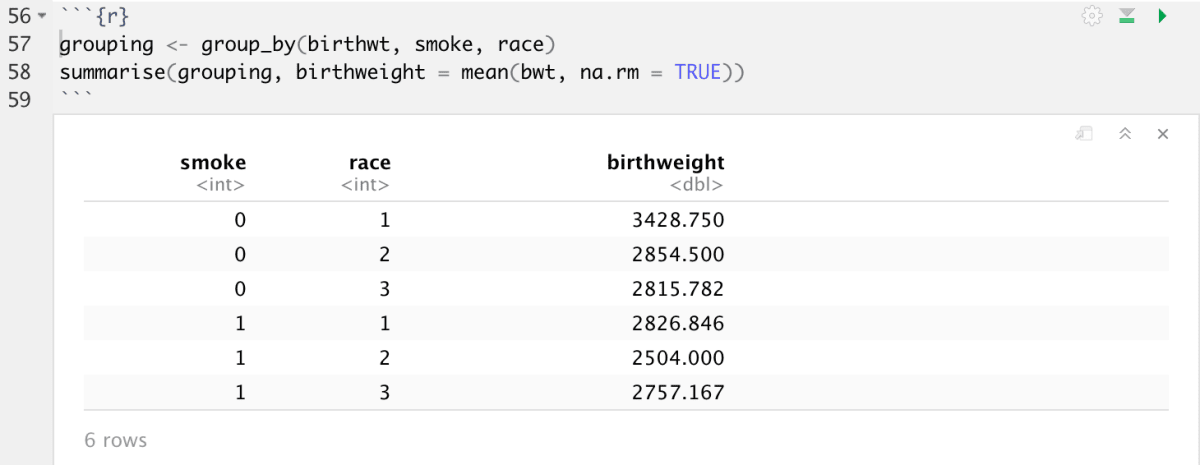We can compare each of the means of different groups from this table. E.g. we can compare the effect of smoking on race 1.

Together `group_by()` and `summarise()` provide one of the tools that you’ll use most commonly when working with dplyr: grouped summaries.